1597407660

# The mathmatics of the perceptron

I have been learning about machine learning for some time now and I believe that, to really understand something, one must understand the basics. In this blog post I will go through the theory and at the end I’ll share the code for a perceptron, the most basic neural network there is that dates back to 1958 created by Frank Rosenblatt.

To train and test it, I’ll use a fragment of the mnist dataset. This dataset contains images of 28 x 28 pixels of hand-written digits from 0 to 9. We’ll try to classify two, four and six digits and see that it becomes increasingly more difficult to make predictions.

The concept of the perceptron is that, with only a simple layer of calculations, our code can understand rules and paths without explicitlly writing them.

Let’s go through the theory, I’ll explain it using only two digits (classes), 0 and 1:

Let’s start by laying some important matrices. The matrix of weights (W) and biases (B) transform the matrix of inputs (X) into the net inputs (Z) and then, an activation function transforms Z into activation outputs or predictions (A). In this case, the input matrix’s rows are all the samples (the vectorized mnist images) and the columns are all the pixel values (we’ll start by using only the images of 0s and 1s). Visually, what we have is this: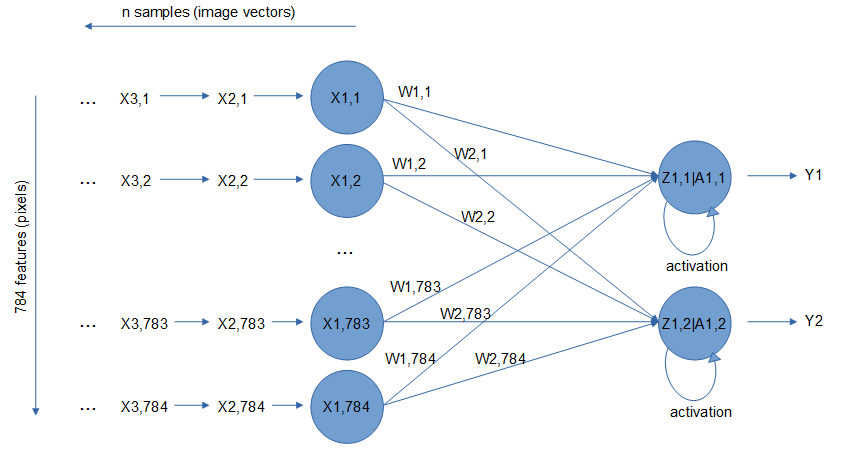Note that in the image X is transposed for an easier understanding.

For each vectorized image we’ll get a pair of predictions (either it is a 0 or a 1) based on the parameters (weights and biases), this process is called the forward propagation. The calculation is the following: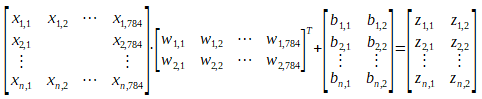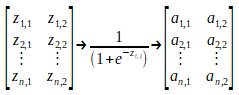To make sure there are no errors when calculating the net input, the dimensionality of all matrixes should match. So, Z of dimensions n x 2, being n the number of samples and 2 how many classes we want to predict, is calculated with the dot product of X of dimensions n x 784 (28 x 28 pixel images vectorized) and W of dimension 2 x 784 transposed: (n x 784) dot (784 x 2). The inner dimensions match, and the resulting matrix is of shape n x 2 that, added to B with the same shape n x 2, make the Z matrix.

Now we activate Z with an element-wise calculation to obtain A of dimensions still n x 2, meaning that for all the n vectorized images there are two possible predictions (1 or 0) and the greatest is chosen as the predicted value.

#perceptron #forward-propagation #machine-learning #backpropagation #deep learning

## Buddha Community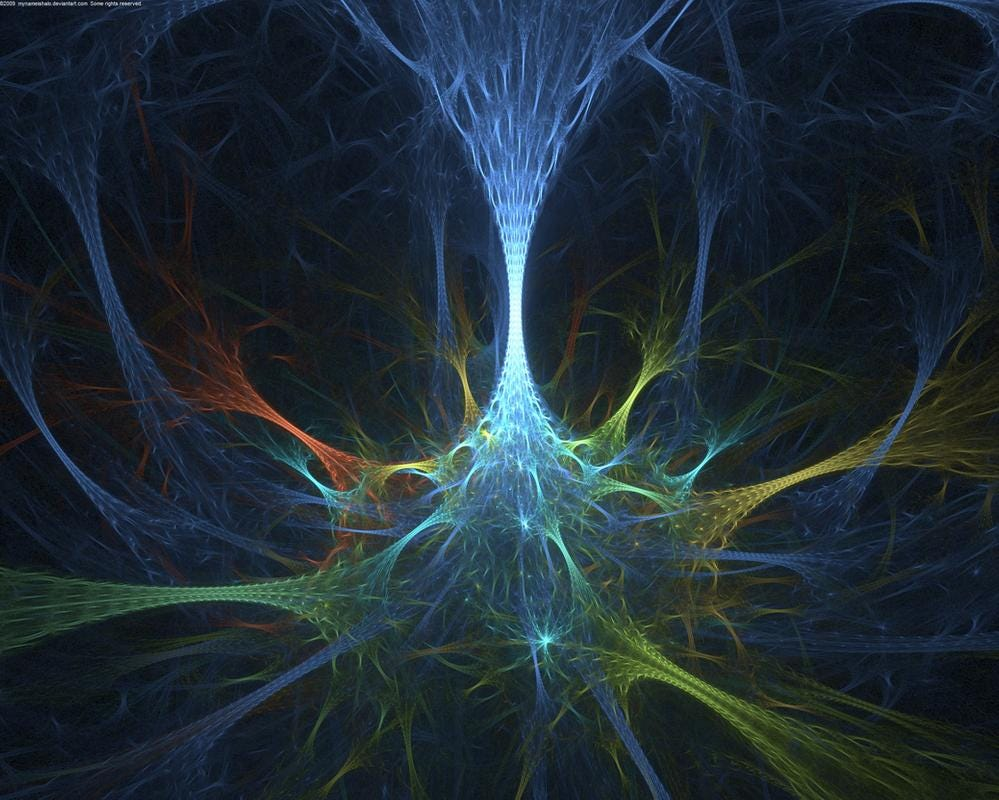1597407660

## The mathmatics of the perceptron

I have been learning about machine learning for some time now and I believe that, to really understand something, one must understand the basics. In this blog post I will go through the theory and at the end I’ll share the code for a perceptron, the most basic neural network there is that dates back to 1958 created by Frank Rosenblatt.

To train and test it, I’ll use a fragment of the mnist dataset. This dataset contains images of 28 x 28 pixels of hand-written digits from 0 to 9. We’ll try to classify two, four and six digits and see that it becomes increasingly more difficult to make predictions.

The concept of the perceptron is that, with only a simple layer of calculations, our code can understand rules and paths without explicitlly writing them.

Let’s go through the theory, I’ll explain it using only two digits (classes), 0 and 1:

Let’s start by laying some important matrices. The matrix of weights (W) and biases (B) transform the matrix of inputs (X) into the net inputs (Z) and then, an activation function transforms Z into activation outputs or predictions (A). In this case, the input matrix’s rows are all the samples (the vectorized mnist images) and the columns are all the pixel values (we’ll start by using only the images of 0s and 1s). Visually, what we have is this:Note that in the image X is transposed for an easier understanding.

For each vectorized image we’ll get a pair of predictions (either it is a 0 or a 1) based on the parameters (weights and biases), this process is called the forward propagation. The calculation is the following:To make sure there are no errors when calculating the net input, the dimensionality of all matrixes should match. So, Z of dimensions n x 2, being n the number of samples and 2 how many classes we want to predict, is calculated with the dot product of X of dimensions n x 784 (28 x 28 pixel images vectorized) and W of dimension 2 x 784 transposed: (n x 784) dot (784 x 2). The inner dimensions match, and the resulting matrix is of shape n x 2 that, added to B with the same shape n x 2, make the Z matrix.

Now we activate Z with an element-wise calculation to obtain A of dimensions still n x 2, meaning that for all the n vectorized images there are two possible predictions (1 or 0) and the greatest is chosen as the predicted value.

#perceptron #forward-propagation #machine-learning #backpropagation #deep learning1593486900

## (Part 2) Pattern Recognition and Perceptrons

Welcome! After completing our journey to station number 1, we are now about to begin our ride to the second station. If you need assistance, please click on either of the links: FacebookLinkedInInstagram_Quora _to contact the driver. Get your tickets of ‘time’ and we will start!Special thanks to @Igot7Linn on Twitter and @art.soopified on Instagram.

Nature uses only the longest threads to weave her patterns so that each small piece of her fabric reveals the organization of the entire tapestry.

-Richard Feynamnn

The more we explore those tapestries, the more fascinating the world around us seems. One such example would be the Fibonacci sequence.Fibonacci Spiral observed in a seashell

But there lie more fascinating patterns that may not be as simple. And we humans are very much curious about recognizing them.

Consider the case of Iris Flowers. (Beautiful! Aren’t they?)Iris Flowers

A British statistician and geneticist, Ronald Fisher used a dataset of these flowers ( which was collected by Dr. Edgar Anderson) to classify three types of iris flowers (Iris setosa, virginica, and Versicolor) using the widths and lengths of their petals and sepals. Basically he was looking for a pattern among the _features _(widths and lengths of petals and sepals) that would allow him to link that pattern to the respective class or type of flower(Iris Setosa, Virginica, and Versicolor). He evaluated magical (statistical) parameters to recognize which pattern belonged to which class. But what precisely did he do for that? He used the technique of Linear Discriminant Analysis. I’d recommend to read out his paper if you want to know more.

With this, let me introduce a few definitions:

1. pattern is an arrangement of features.
2. pattern class is a family of patterns that share some common properties.
3. Pattern recognition by machine involves techniques for assigning patterns to their respective classes-automatically and with as little human intervention as possible.
4. The common pattern arrangements used in practice are vectors. Pattern vectors are represented as: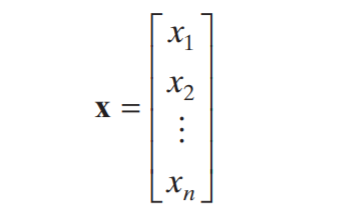where each component _xᵢ _isrepresented as the iₜₕ feature and _n _is the total number of such features associated with the pattern.

In the case of Iris Dataset, the pattern vector consists of four elements (length and the width of sepal and petal). The three pattern classes correspond to the varieties setosa, virginica, and versicolor.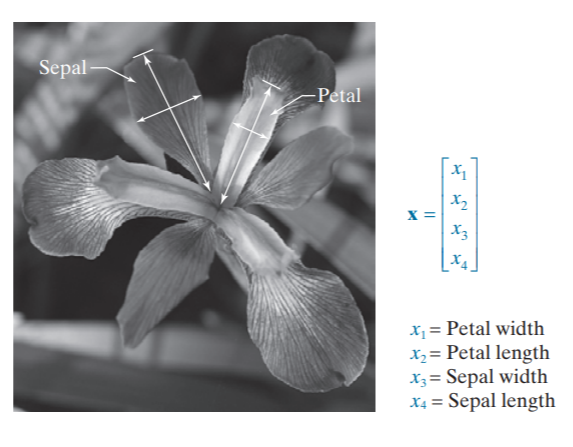Petal and sepal width and length measurements (see arrows) performed on iris flowers for the purpose of data classification. The image shown is of the Iris virginica class.

Now, allow me to present you with a beautiful plot from the Iris Dataset. For simplicity, I have plotted only 2 features: sepal length and petal length for 2 classes: setosa and versicolor.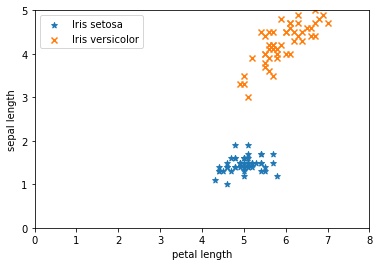The graph plotted for 2 classes: Iris Setosa and Iris Versicolor of Iris Dataset.

Let us say I want a device(algorithm) that learns the pattern of these two classes and provides me with a boundary that separates the two classes. The boundary would essentially represent an equation of a line. But how do I find that equation? For this let us understand a perceptron.

# Perceptron

In essence, a perceptron learns a linear boundary between two linearly separable pattern classes We will consider the perceptron model for two pattern classes.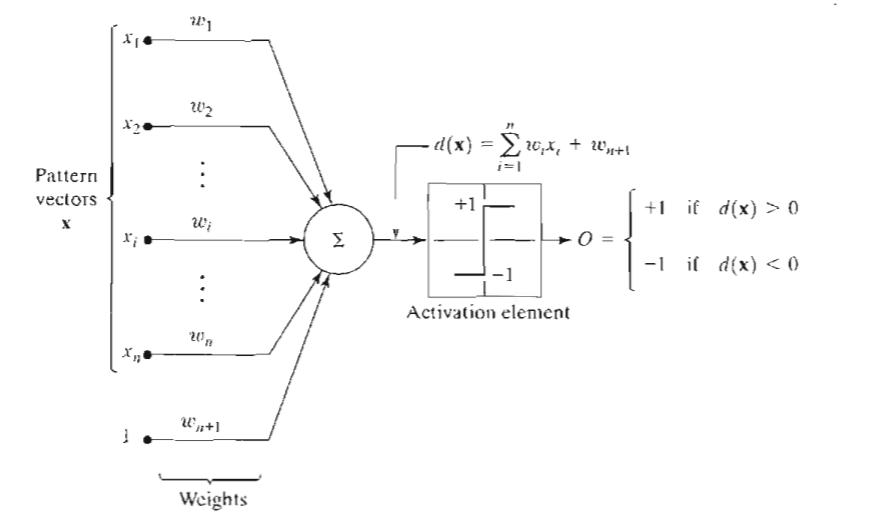Fig. 1 Perceptron Model for two pattern classes

The output of this device is based on a weighted sum of its inputs; that is,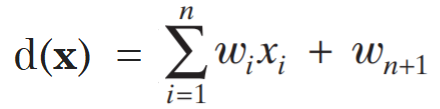which is a linear function with respect to the components of the pattern vectors? The coefficients ωᵢ , i= 1,2,. . . , n, n + 1 called weights, modify the inputs before they are summed and fed into the threshold element. The last weight that is not multiplied by any coefficient is often referred to as the bias. The function that maps the output of the summing junction into the final output of the device sometimes is called the activation function.

The equation for the decision boundary that separates the two datasets will be obtained by equating d(x) to zero: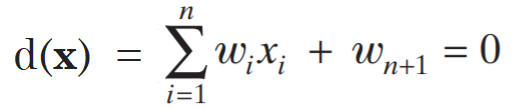or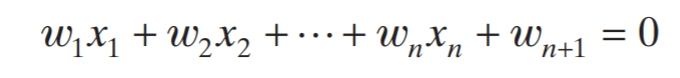or in vector form as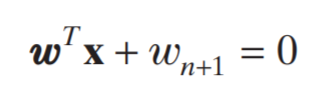where** w **and x are n-dimensional column vectors and the first term is the dot (inner) product of the two vecto

#iris-dataset #object-detection #pattern-recognition #perceptron-algorithm #perceptron #algorithms1596826380

# Preface

In part 1 of this series (Linear Equation as a Neural Network building block) we saw what linear equations are and also had a glimpse of their importance in building neural nets.

In part 2 (Linear equation in multidimensional space) we saw how to work with linear equations in vector space, facilitating us to work with many variables.

Now I will show you how one linear equation can be embedded into another one (mathematically this is known as Function Composition) to structure a neural network. I will then proceed with how linear combination of weight matrices and feature vectors can help us with all the maths involved in the feed forward pass, ending this story with a working example.

This series has a mantra (note of comfort) that I will repeat below in case the eventual reader hasn’t read part 1.

# Mantra: A note of comfort to the eventual reader

I won’t let concepts like gradient and gradient descent, calculus and multivariate calculus, derivatives, chain rule, linear algebra, linear combination and linear equation become boulders blocking your path to understanding the math required to master neural networks. By the end of this series, hopefully, these concepts will be perceived by the reader as the powerful tools they are and how they are simply applied to building neural networks.

# Function composition

If linear equations are neural networks’s building blocks, function composition is what binds them. Great! But what is function composition?

Lets consider the 2 linear equations below: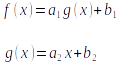Equation 1: Function composition

What is different here? The difference is that in order to calculate the value of f(x), for any given**_ x_**, we first need to compute the value of g(x). This simple concept is known as function composition.

The above definition and notation, although correct, are not the ones commonly used. Function composition is normally defined as an operation where the result of function g(x) is applied to function f(x), yielding in a function h(x). Thus: h(x) = f(g(x)). **Another notationis: **(f ∘g)(x)=f(g(x)). And the above equations are written as follows: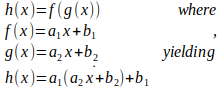Equation 2: h(x) written as a composition of f(x) and g(x)

Schematically the above function composition can drawn as illustrated in figure 1.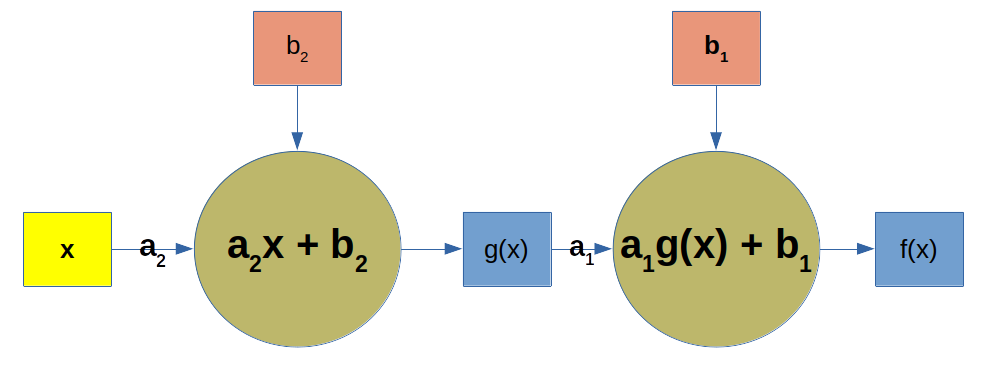Figure 1: Function composition described as a network

In the above picture x is the input, or the independent variable. This input is multiplied by the angular coefficient _a_₂ which added to _b_₂ yields g(x). In turn, g(x) is multiplied by **_a₁, _**then added to b₁ resulting in f(x).

I find this really cool! Aren’t we getting closer to a neural network?

Believe me. If you understood what these two concepts (linear equation and function composition) are, you understood mathematically 80% of what a feed forward neural network is. What remains is to understand how to add additional independent variables (x₁x₂, …, xₙ) to enable our neural network to work with many (probably the majority) of real world problems one deals with.

#neural-networks #multilayer-perceptron #feed-forward #perceptron #neural networks1602954000

## What is a Perceptron? – Basics of Neural Networks

A single-layer perceptron is the basic unit of a neural network. A perceptron consists of input values, weights and a bias, a weighted sum and activation function.

In the last decade, we have witnessed an explosion in machine learning technology. From personalized social media feeds to algorithms that can remove objects from videos. Like a lot of other self-learners, I have decided it was my turn to get my feet wet in the world of AI. Recently, I decided to start my journey by taking a course on Udacity called, Deep Learning with PyTorch. Naturally, this article is inspired by the course and I highly recommend you check it out!

If you have taken the course, or read anything about neural networks one of the first concepts you will probably hear about is the perceptron. But what is a perceptron and why is it used? How does it work? What is the history behind it? In this post, we will briefly address each of these questions.

## A little bit of history

The perceptron was first introduced by American psychologist, Frank Rosenblatt in 1957 at Cornell Aeronautical Laboratory (here is a link to the original paper if you are interested). Rosenblatt was heavily inspired by the biological neuron and its ability to learn. Rosenblatt’s perceptron consists of one or more inputs, a processor, and only one output.

#machine-learning #neural-networks #deep-learning #perceptron #artificial-intelligence1594203180

## Fundamental Concepts of Neural Nets: Perceptron

Neural Networks are extremely efficient tools for analyzing past and present data. They are made such that they reflect a biological network of neurons at a very fundamental level. However, even this fundamental image of our mind has done wonders with the help of computation resources like high processing power and voluminous storage systems; and with each passing day, we are getting nearer to building closer representations of the mind. The amalgamation of the human mind and external machines has the capability to not only change the world we live in, but also create new ones. Decades or even centuries of research goes into such initiatives, but here also comes the question of mass extinction before the achievement of something so close to a miracle.
Coming back to basics, the study of neural networks is called Deep Learning and it expands into several interesting sub-topics.

#neural-networks #data-science #perceptron #fundamental #asp.net (.net) #.net